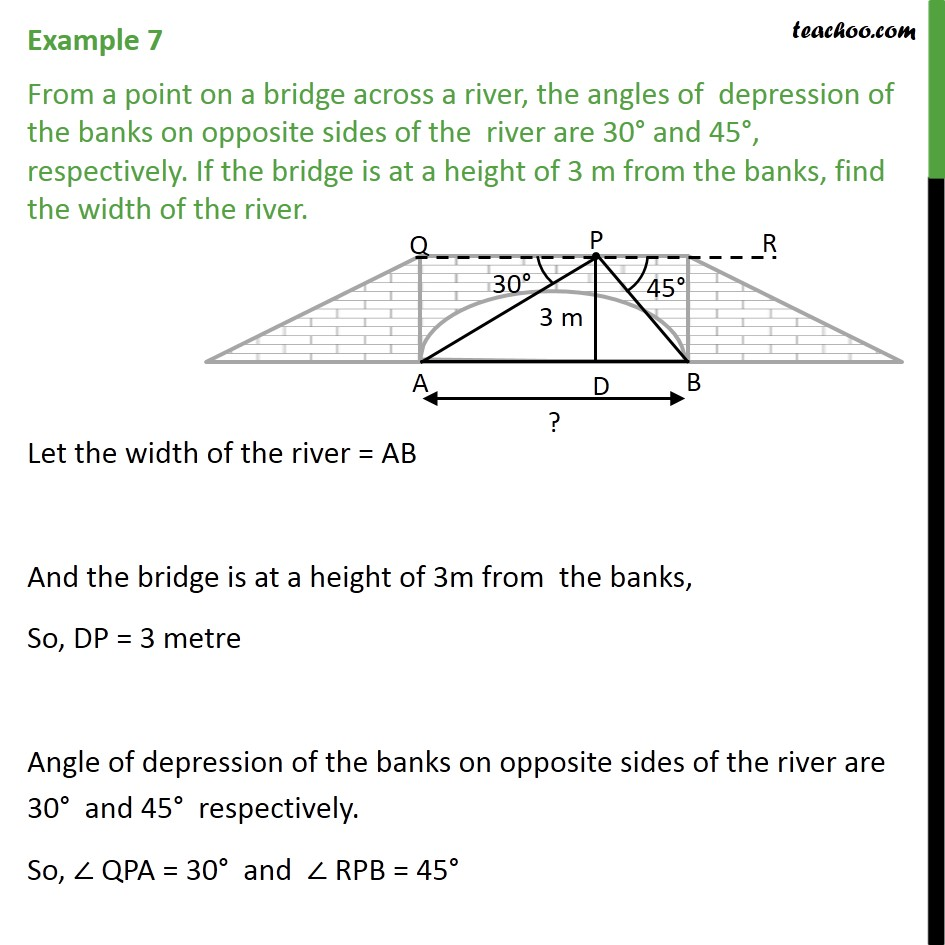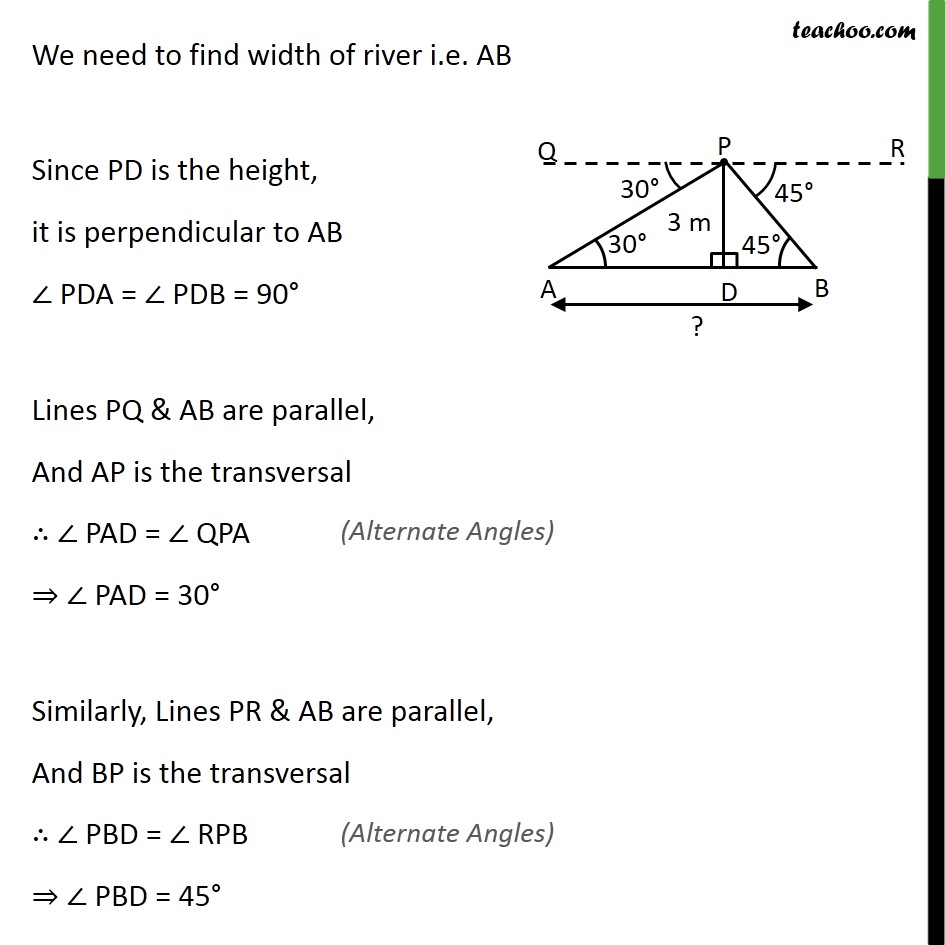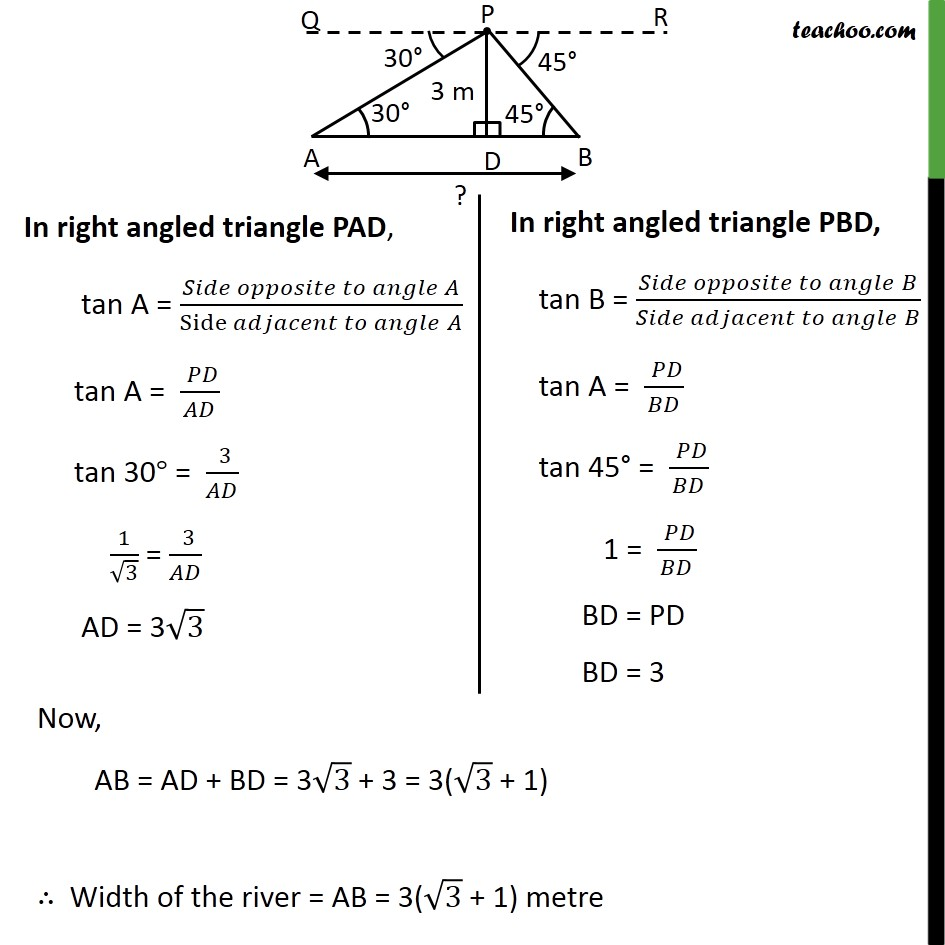1. Chapter 9 Class 10 Some Applications of Trigonometry (Term 2)
2. Concept wise
3. Questions easy to difficult

Transcript

Example 7 From a point on a bridge across a river, the angles of depression of the banks on opposite sides of the river are 30 and 45 , respectively. If the bridge is at a height of 3 m from the banks, find the width of the river. Let the width of the river = AB And the bridge is at a height of 3m from the banks, So, DP = 3 metre Angle of depression of the banks on opposite sides of the river are 30 and 45 respectively. So, QPA = 30 and RPB = 45 We need to find width of river i.e. AB Since PD is the height, it is perpendicular to AB PDA = PDB = 90 Lines PQ & AB are parallel, And AP is the transversal PAD = QPA PAD = 30 Similarly, Lines PR & AB are parallel, And BP is the transversal PBD = RPB PBD = 45 Now, AB = AD + BD = 3 3 + 3 = 3( 3 + 1) Width of the river = AB = 3( 3 + 1) metre